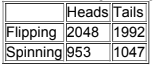×
Get Full Access to Elementary Statistics - 12 Edition - Chapter 11.3 - Problem 5 bsc
Get Full Access to Elementary Statistics - 12 Edition - Chapter 11.3 - Problem 5 bsc

×

# In Exercise, test the given claim.Denomination Effect In aISBN: 9780321836960 18

## Solution for problem 5 BSC Chapter 11.3

Elementary Statistics | 12th Edition

• Textbook Solutions
• 2901 Step-by-step solutions solved by professors and subject experts
• Get 24/7 help from StudySoup virtual teaching assistantsElementary Statistics | 12th Edition

4 5 1 307 Reviews
27
1
Problem 5 BSC

Flipping and Spinning Pennies Use the data in the table below with a 0.05 significance level to test the claim that when flipping or spinning a penny, the outcome is independent of whether the penny was flipped or spun. (The data are from experimental results given in Chance News.) Does the conclusion change if the significance level is changed to 0.01?Step-by-Step Solution:
Step 1 of 3

Solution  5BSC

As per the given information we have P value is 0.0648. On comparing this with significance level we find that. Thus we fail to reject the claim that the form of 100 Yuan is independent of whether the money was spent. Or we can say that we do not have sufficient evidence that to accept the claim of denomination effect.

Step 2 of 3

Step 3 of 3

##### ISBN: 9780321836960

This full solution covers the following key subjects: Yuan, given, Money, Women, denomination. This expansive textbook survival guide covers 121 chapters, and 3629 solutions. Since the solution to 5 BSC from 11.3 chapter was answered, more than 412 students have viewed the full step-by-step answer. This textbook survival guide was created for the textbook: Elementary Statistics, edition: 12. Elementary Statistics was written by and is associated to the ISBN: 9780321836960. The answer to “?Flipping and Spinning Pennies Use the data in the table below with a 0.05 significance level to test the claim that when flipping or spinning a penny, the outcome is independent of whether the penny was flipped or spun. (The data are from experimental results given in Chance News.) Does the conclusion change if the significance level is changed to 0.01?” is broken down into a number of easy to follow steps, and 61 words. The full step-by-step solution to problem: 5 BSC from chapter: 11.3 was answered by , our top Statistics solution expert on 03/15/17, 10:30PM.

Unlock Textbook Solution